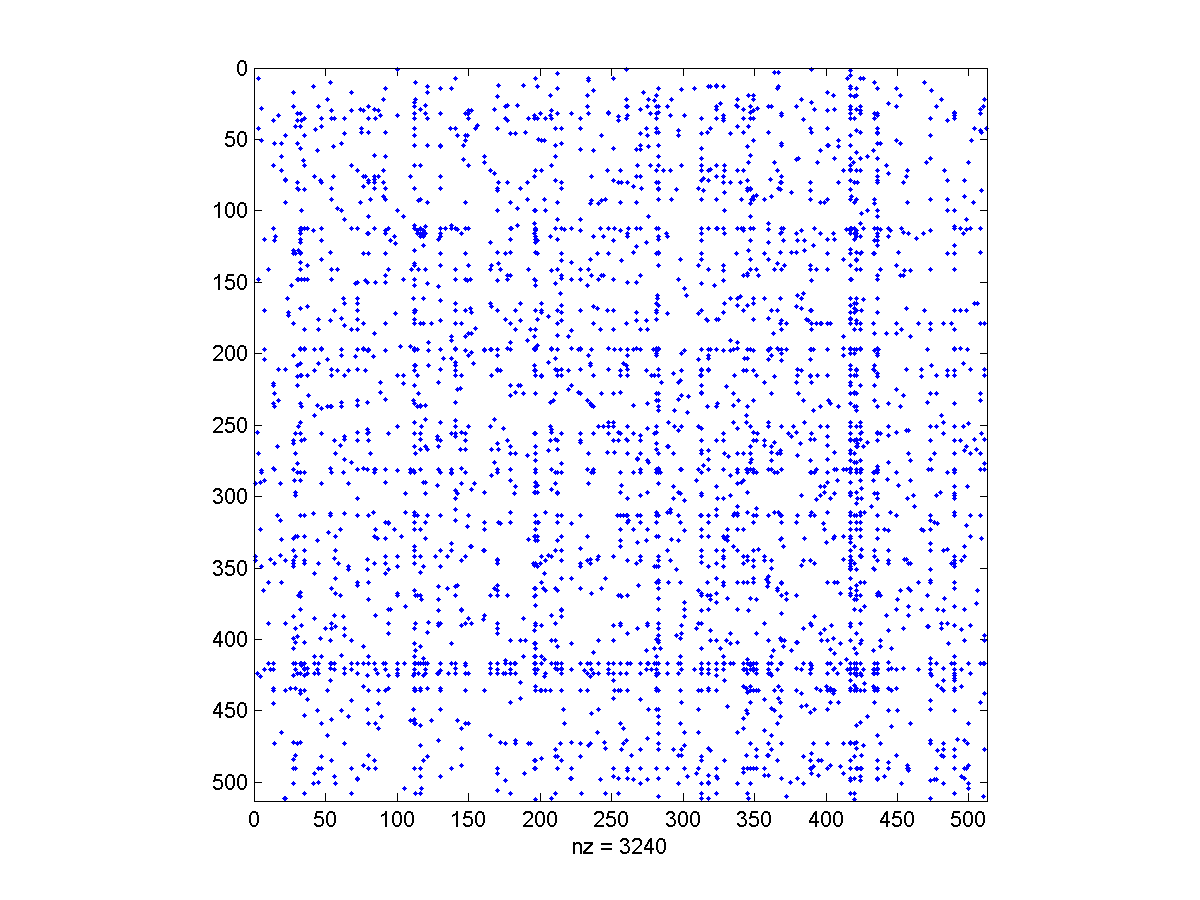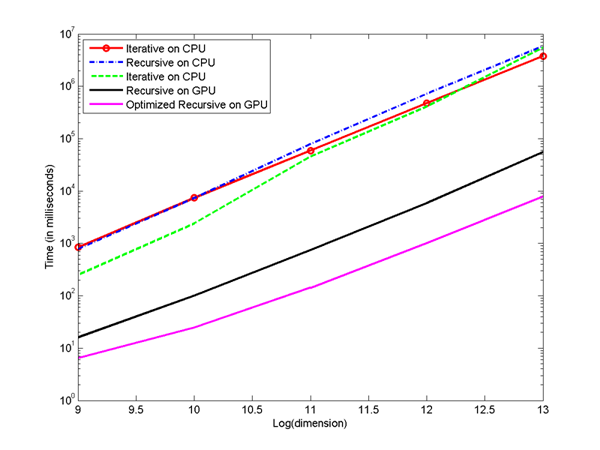An early talk (slides) given at PMAA'08 .

Preprint accepted to Parallel Computing, Solving Path Problems on the GPU (pdf)Host Code
Device Code
Header file (constants & parameters)
C++ code (for verification)
The input format is a file of m+1 (# edges + 1) lines where each line is
"from to weight",
except for the first header line which is of the form
"n n m"
for n=# of vertices and m=# edges.
An example is here for a 4096 vertex graph with 28567 edges (rename it if needed).